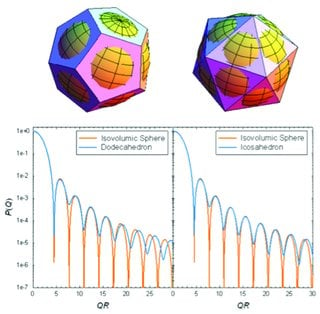# Scattering functions of Platonic solids

## J. Appl. Cryst. (2011). 44, 545-557 (doi.org/10.1107/S0021889811011691)The small-angle scattering form factors S(Q) of dodecahedron (left), icosahedron (right) and the isovolumetric hard sphere.

Platonic solids, including tetrahedra, hexahedra, octahedra, dodecaheda and icosahedra, form an essential basis for the topology of condensed matter in a variety of contexts. For each given geometry, the Debye spatial autocorrelation function γ(r), the pair distance distribution function p(r) and the intraparticle structure factor (form factor) S(Q) are calculated analytically and compared with the corresponding scattering functions of a spherical reference system with the same particle volume. As an illustrative example, the quantitative interpretation of small-angle scattering data from an icosahedral virus is presented.

X. Li, C.-Y. Shew, L. He, F. Meilleur, D. A. A. Myles, E. Liu, Y. Zhang, G. S. Smith, K. W. Herwig, R. Pynn and W.-R. Chen